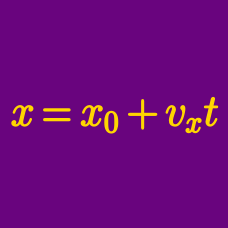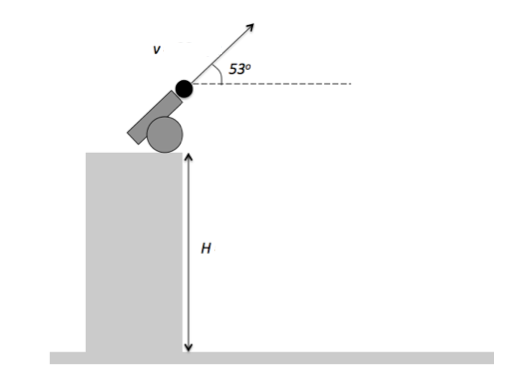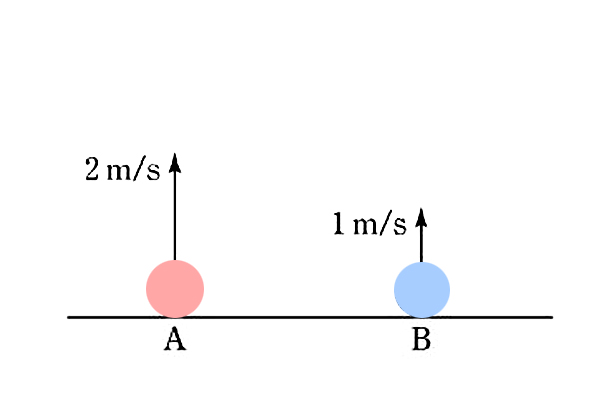Classical Mechanics

# Projectile motion - intermediate

A $300 \text{ g}$ football is kicked with an initial velocity of $140 \text{ m/s}$ in a direction that makes a $30^\circ$ angle with the horizon. Find the peak height of the football.

Gravitational acceleration is $g = 10 \text{ m/s}^2,$ and air resistance is negligible.The figure above depicts a cannon on the edge of a $H= 73 \text{ m}$ high cliff. The cannon fires a cannonball at a $53 ^\circ$ angle with the horizon at a speed of $25 \text{ m/s} .$ If the height of the cannon itself is negligible, what is the maximum height from the ground that the cannonball reaches?

The gravitational acceleration is $g = 10 \text{ m/s}^2,$ and $\sin{53^\circ}$ and $\cos{53^\circ}$ are about $0.8$ and $0.6,$ respectively.

A projectile fired from the ground has a maximum range of $132 \text{ m}.$ What is the maximum height attained by it?

Assume that air resistance is negligible and gravitational acceleration is $g= 10 \text{ m/s}^2.$

A man kicks a $300 \text{ g}$ ball from the ground in a direction that makes a $45^\circ$ angle with the horizon. He intends to make the ball land on top of a moving truck. If the initial horizontal distance between the truck and the ball, at the instant of the kick, is $d_o = 36 \text{ m},$ and the truck moves directly away from the ball with a velocity of $v = 9 \sqrt{2} \text{ m/s},$ in what magnitude of velocity $v_o$ should the ball be kicked in order to make it land on the truck?

The height of the truck and air resistance are both negligible, and the gravitational acceleration is $g =10 \text{ m/s}^2.$Two identical marbles $A$ and $B$ are thrown vertically from the ground with speeds of $2 \text{ m/s}$ and $1 \text{ m/s},$ respectively, as shown above. Let $a$ be the distance traveled by $A$ until it returns back to the ground. Let $b$ be the distance traveled by $B$ until it returns back to the ground. What is $\displaystyle{\frac{a}{b}?}$

×